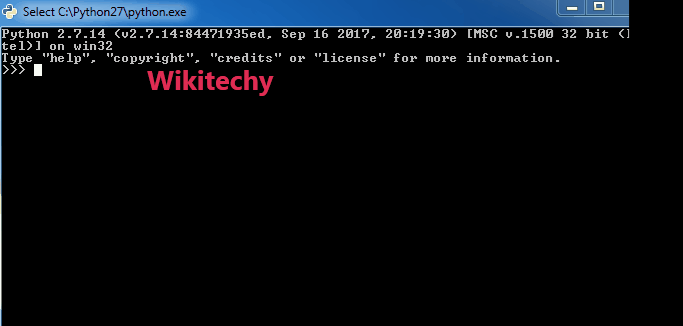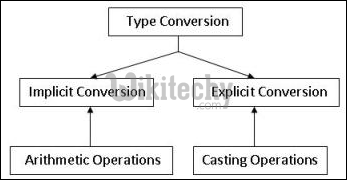# python tutorial - Type Conversion | Type Conversion in Python - learn python - python programmingLearn Python - Python tutorial - interesting-facts-about-strings-python conversion - Python examples - Python programs

Python defines type conversion functions to directly convert one data type to another which is useful in day to day and competitive programming. This article is aimed at providing the information about certain conversion functions.1. int(a,base) : This function converts any data type to integer. ‘Base’ specifies the base in which string is if data type is string.

2. float() : This function is used to convert any data type to a floating point number
.

python tutorial - Output :

3. ord() : This function is used to convert a character to integer.

4. hex() : This function is to convert integer to hexadecimal string.

5. oct() : This function is to convert integer to octal string.

python - Sample - python code :

python tutorial - Output :

6. tuple() : This function is used to convert to a tuple.

7. set() : This function returns the type after converting to set.

8. list() : This function is used to convert any data type to a list type.

python - Sample - python code :

python tutorial - Output :

9. dict() : This function is used to convert a tuple of order (key,value) into a dictionary.

10. str() : Used to convert integer into a string.

11. complex(real,imag) : : This function converts real numbers to complex(real,imag) number.

python - Sample - python code :

python tutorial - Output :

Wikitechy tutorial site provides you all the learn python , python programming online training , python course online , python programming course